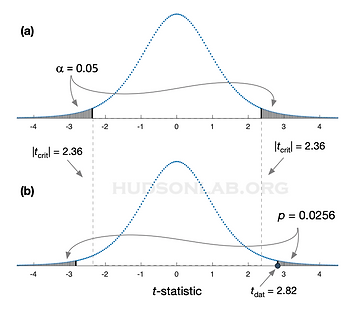top of page

#### A Beginners Guide to Student's t-test

• Under what circumstances is the t-test used?

• What is the logic of Student's t-test?

• How is the t-test computed and interpreted?

• When does the t-test allow us to compare and/or test hypotheses?Under what circumstances is the t-test used?

Student's t-test is a statistical procedure that is used in two ways:

1. to determine whether a mean value differs from a theoretically predicted value

2. to determine whether a mean value differs from a second mean value

These two uses of the t-test have slightly different implementations, although the logic is the same in both. For this reason, I will only detail the mathematics used in the first case, but be aware that the logic and interpretation are identical in both.

In the first case, you want to test the null hypothesis which states that the underlying signal, s, is:

s =      {   = const

and you collect a single dataset described by:

D = s + noise

In the second case, you collect two datasets to test the null hypothesis which states that the underlying signal responsible for both datasets have the same mean value:

s1 =

s2 =

and the two datasets are described by:

D1 = s1 + noise1

D2 = s2 + noise2

In both cases, the t-test is meant to determine whether the null hypothesis, H0, described by these equations can be rejected.

What is the logic of Student's t-test?

Student's t-test is perhaps the standard, and simplest, of the statistical hypothesis tests

​It follows the basic logic that:

• An underlying signal that conforms to H0 will produce data that is relatively close to mu.

• If the computed (observed) mean value of the dataset is 'too far' from the predicted value, mu, it is evidence against the null hypothesis.

How is the t-test computed and interpreted?

To determine if the value of the observed data mean is sufficiently far from the theoretically predicted value, mu, you:

1. Assume that the null hypothesis, H0, is correct

2. From this assumption, compute the sampling distribution of the t-statistic (Fig. 1)

3. Compute the p-value associated with the observed t-statistic​

4. If this p-value is below your pre-set alpha criterion, reject H0

In the example shown in Fig. 1, we assume there is an observed dataset, and these data differ from the predicted value of    by the following amounts: = [1.1, 2, 0, 0, 0.4, 0.6, 3, 1.2].

The t-statistic is defined as:

We can then compute the t-statistic with the following lines of Matlab code:

>> D=[1.1, 2, 0, 0, .4, .6,3, 1.2];

>> [h,p,ci,stats]=ttest(D); stats.tstat

Further, the probability mass contained by t-values larger than this t-statistic is part of the previous Matlab output, and can be retrieved by typing:

>> p

which in this case is p = ​0.0256.

• Notice that the t-statistic is monotonic with the difference between the mean of your dataset and the predicted value of the underlying signal,

• The t-statistic is used to determine whether the observed data are 'too far' from the predictions of H0 to warrant rejecting the null.

The plots in Fig. 1 make it clear that the t-statistic, t = 2.8 is greater than the criterion, tcrit = 2.4, and therefore this corresponds to a ‘statistically significant’ statistical hypothesis test​

• In other words, we reject H0

• It is further from the predictions of H0 than the threshold value of t      defined by    .

When does the t-test allow us to compare and/or test hypotheses?

There is some subtlety in the transition between measuring the separation between the data mean and predicted signal value,                   , and the determination that there is a statistically significant difference between the data and the predictions of H0

In particular, measurements and a Hypothesis tests are distinct methods with quite different computations, so it is important to see to what extent the t-test constitutes an hypothesis test and not simply a measurement.

• The key to understanding the difference is to look at the computations being performed

• A measurement is based on a single probability distribution that tells us the most likely values of the underlying signal,

• A hypothesis test (model comparison) is based on the likelihood of the hypothesis being correct, which requires that the likelihoods (or probabilities) of competing hypotheses be computed and compared.

• In both cases, the dataset (D) is the important information that provides evidence for the computation​

• In the case of a measurement, that computation uses the data mean to compute the likelihood over underlying signal values

• This is very similar to the procedure used to compute a sampling distribution, the basis for the t-test, in that both rely on a single model of the data [here, d =    + noise] for their computation​s

• In the case of hypothesis testing, that computation uses the data mean to compute the likelihood function over possible hypotheses​, where each hypothesis posits a different model of the data [e.g., d = mu + noise vs. d = mu + betax + noise, etc.]

• Reliance on sampling distributions introduces a number of weaknesses in the t-test, including:​

• lack of an ability to differentiate among competing alternative hypotheses​

• no ability for experiments to provide evidence favoring any hypothesis (null or otherwise)Fig. 1critbottom of page